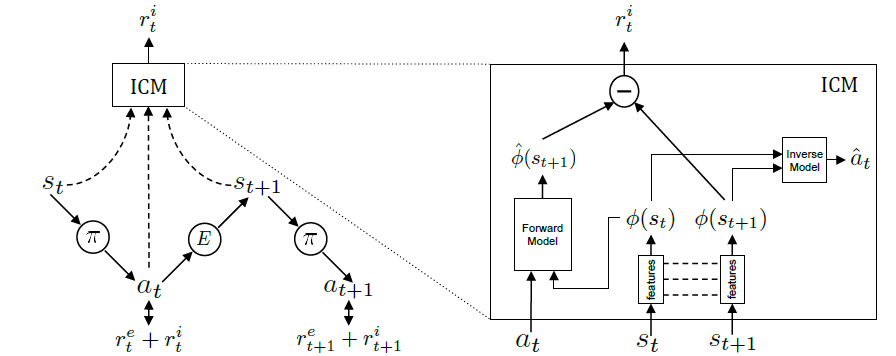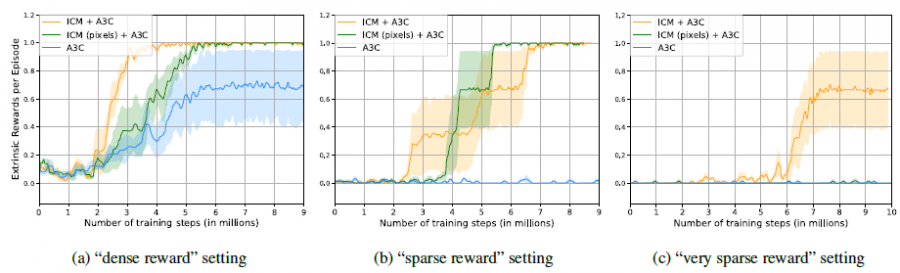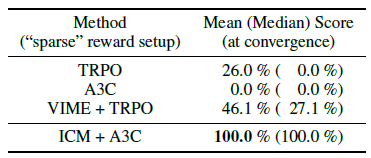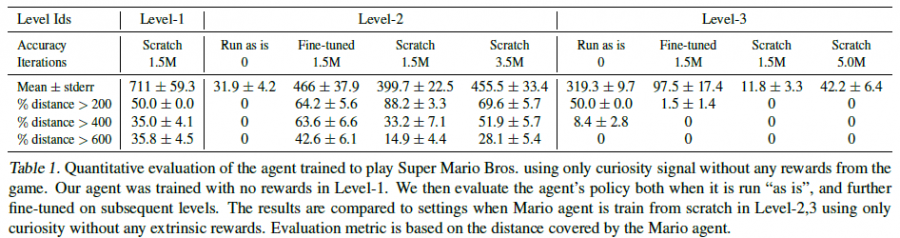# 单智能体强化学习算法

## ICM：Curiosity-Driven Exploration by Self-Supervised Prediction

Posted by MY on August 5, 2020

## 一、问题

• 激励agent去探索更多的新状态（novel state）；
• 激励agent去执行一些会减少自己对于未来不确定性的动作，或者可以反过来理解为去执行一些能增加自己对于未来的信息的动作。

## 二、解法

ICM框架如下图所示：$\max _{\theta_{P}} \mathbb{E}_{\pi\left(s_{t} ; \theta_{P}\right)}\left[\Sigma_{t} r_{t}\right]$

### 预测误差作为intrinsic reward

• agent可以控制，比如agent开枪射出子弹
• agent不可以控制的，但是对agent有实际影响的，比如怪物的移动
• 本质上无效的信息，比如agent走着走着，从白天变成黑夜，背景变黑了

### Self-supervised prediction for exploration

#### Inverse Model提取state表征

• 建立模型根据当前state $s_{t}$和下一个state $s_{t+1}$预测中间采取的动作：$\hat{a}_{t}=g\left(s_{t}, s_{t+1} ; \theta_{I}\right)$
• 最小化预测动作与真实动作的loss：$\min_{\theta_{I}} L_{I}\left(\hat{a}_{t}, a_{t}\right)$

#### Forward Model预测下一时刻state表征

• 预测$t+1$时的state encoding：$\hat{\phi}\left(s_{t+1}\right)=f\left(\phi\left(s_{t}\right), a_{t} ; \theta_{F}\right)$
• 最小化预测状态encoding与真实状态encoding之间的loss：$L_{F}\left(\phi\left(s_{t}\right), \hat{\phi}\left(s_{t+1}\right)\right)=\frac{1}{2}\left|\hat{\phi}\left(s_{t+1}\right)-\phi\left(s_{t+1}\right)\right|_{2}^{2}$
• 计算intrinsic reward：$r_{t}^{i}=\frac{\eta}{2}\left|\hat{\phi}\left(s_{t+1}\right)-\phi\left(s_{t+1}\right)\right|_{2}^{2}$

$\min _{\theta_{P}, \theta_{I}, \theta_{F}}\left[-\lambda \mathbb{E}_{\pi\left(s_{t} ; \theta_{P}\right)}\left[\Sigma_{t} r_{t}\right]+(1-\beta) L_{I}+\beta L_{F}\right]$

## 三、实验内容## 四、缺点

• Inverse Model的idea应用到其他大规模的问题上效果不好确定。因为并不是每一种环境都可以这样去进行熟悉，在复杂环境上预测state和action可能相当困难
• 对环境的熟悉程度不能仅通过预测state来评判，需要更丰富

## 五、优点

##### Share